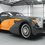# Salute to great Karpekar

The following note I am writing is for real maths lovers i.e. who love to learn new concepts .

I want to write about "Karpekar's Constant"

1) First I would like to mention a simple experiment of playing with numbers.

2) Take any 4-digit number. Eg: Lets take 6384

3) Now arrange this number in its descending order of digits. like: 6384 will be written as 8643... [let this number be 'x']

4) Now arrange the original number in its ascending order of digits. like 6384 will be written as 3468....[let this number be 'y']

5) Now subtract numbers x and y like x-y=8643-3468=5175

6) Now do the same process with the 5175 i.e. arrange in descending order and then in ascending order and then subtract.

7) You will get another number.

8) Do this till you get 6174 as the number.

9) Now if you carry out this process with 6174 then you will observe that in any case this number (6174) remains constant.

10) This number is known as the "Karpekar's Constant".

The specialty of this number is that if you treat any 4-digit number (using at least two different digits) by the above given process then within 7 steps you get the Karpekar's Constant.

If you can construct any sums based on this concept then please write me on atharvabagul2000@gmail.com

Thank YouNote by Atharva Bagul
5 years, 7 months ago

This discussion board is a place to discuss our Daily Challenges and the math and science related to those challenges. Explanations are more than just a solution — they should explain the steps and thinking strategies that you used to obtain the solution. Comments should further the discussion of math and science.

When posting on Brilliant:

• Use the emojis to react to an explanation, whether you're congratulating a job well done , or just really confused .
• Ask specific questions about the challenge or the steps in somebody's explanation. Well-posed questions can add a lot to the discussion, but posting "I don't understand!" doesn't help anyone.
• Try to contribute something new to the discussion, whether it is an extension, generalization or other idea related to the challenge.
• Stay on topic — we're all here to learn more about math and science, not to hear about your favorite get-rich-quick scheme or current world events.

MarkdownAppears as
*italics* or _italics_ italics
**bold** or __bold__ bold
- bulleted- list
• bulleted
• list
1. numbered2. list
1. numbered
2. list
Note: you must add a full line of space before and after lists for them to show up correctly
paragraph 1paragraph 2

paragraph 1

paragraph 2

[example link](https://brilliant.org)example link
> This is a quote
This is a quote
    # I indented these lines
# 4 spaces, and now they show
# up as a code block.

print "hello world"
# I indented these lines
# 4 spaces, and now they show
# up as a code block.

print "hello world"
MathAppears as
Remember to wrap math in $$ ... $$ or $ ... $ to ensure proper formatting.
2 \times 3 $2 \times 3$
2^{34} $2^{34}$
a_{i-1} $a_{i-1}$
\frac{2}{3} $\frac{2}{3}$
\sqrt{2} $\sqrt{2}$
\sum_{i=1}^3 $\sum_{i=1}^3$
\sin \theta $\sin \theta$
\boxed{123} $\boxed{123}$

## Comments

Sort by:

Top Newest
- 5 years, 7 months ago

Log in to reply

Sorry I didn't see that problem but this is the general form popularly known as Karpekar' Constant

- 5 years, 7 months ago

Log in to reply

Even I posted the same thing long time ago.

See this.

- 5 years, 4 months ago

Log in to reply

×

Problem Loading...

Note Loading...

Set Loading...